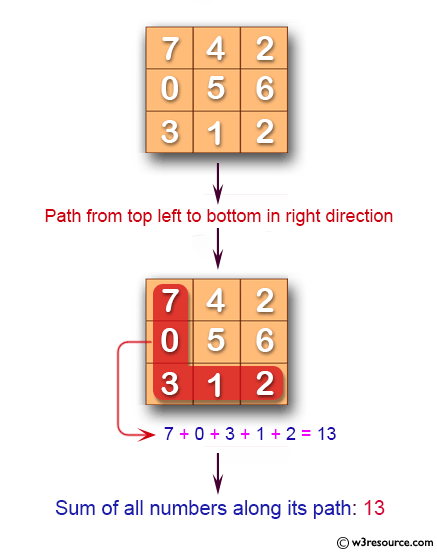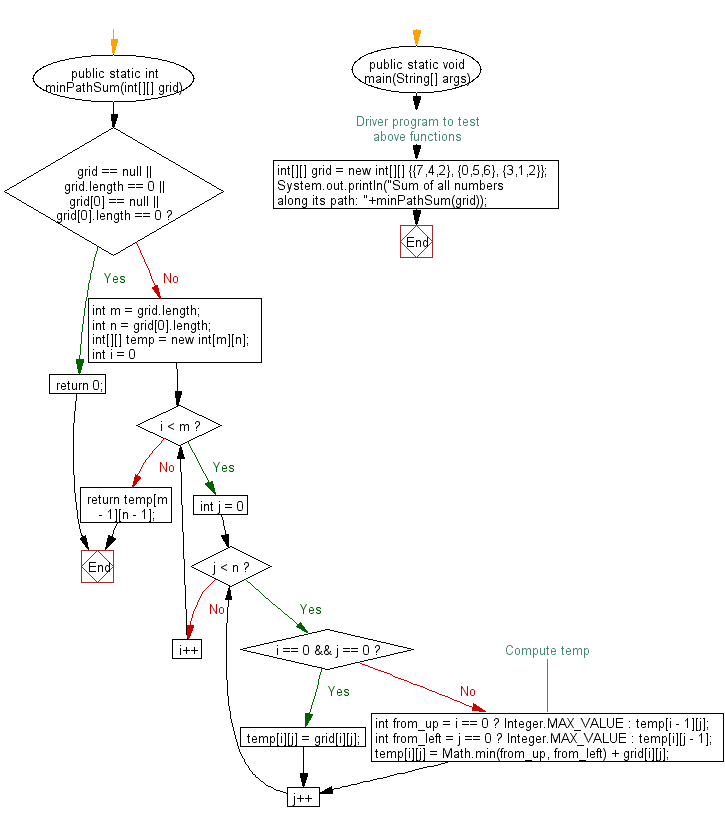﻿ Java: Find a path from top left to bottom in right direction# Java Exercises: Find a path from top left to bottom in right direction

## Java Basic: Exercise-133 with Solution

Write a Java program to find a path from top left to bottom in right direction which minimizes the sum of all numbers along its path.

Note: Move either down or right at any point in time.

Pictorial Presentation:Sample Solution:

Java Code:

``````public class Solution {
public static int minPathSum(int[][] grid) {
if (grid == null || grid.length == 0 || grid == null || grid.length == 0) {
return 0;
}
int m = grid.length;
int n = grid.length;
int[][] temp = new int[m][n];

for (int i = 0; i < m; i++) {
for (int j = 0; j < n; j++) {
if (i == 0 && j == 0) {
temp[i][j] = grid[i][j];
continue;
}
// Compute temp
int from_up = i == 0 ? Integer.MAX_VALUE : temp[i - 1][j];
int from_left = j == 0 ? Integer.MAX_VALUE : temp[i][j - 1];
temp[i][j] = Math.min(from_up, from_left) + grid[i][j];
}
}
return temp[m - 1][n - 1];
}
/* Driver program to test above functions */
public static void main(String[] args) {

int[][] grid = new int[][] {{7,4,2},
{0,5,6},
{3,1,2}};

System.out.println("Sum of all numbers along its path: "+minPathSum(grid));
}
}
```
```

Sample Output:

```Sum of all numbers along its path: 13
```

Flowchart:Java Code Editor:

What is the difficulty level of this exercise?

Test your Programming skills with w3resource's quiz.

﻿

## Java: Tips of the Day

countOccurrences

Counts the occurrences of a value in an array.

Use Arrays.stream().filter().count() to count total number of values that equals the specified value.

```public static long countOccurrences(int[] numbers, int value) {
return Arrays.stream(numbers)
.filter(number -> number == value)
.count();
}
```

Ref: https://bit.ly/3kCAgLb